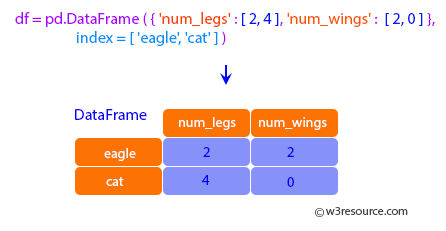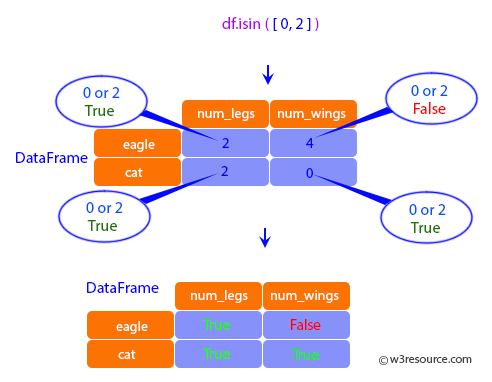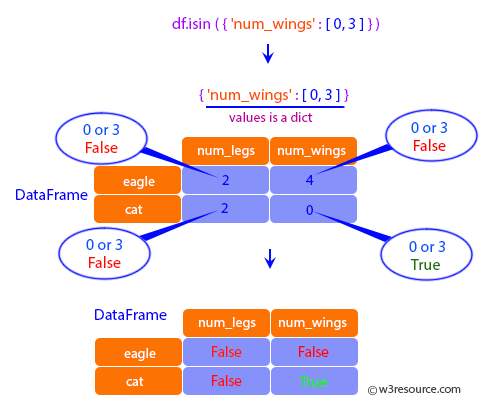Examples

In :
import numpy as np
import pandas as pd

In :
df = pd.DataFrame({'num_legs': [2, 4], 'num_wings': [2, 0]},
index=['eagle', 'cat'])
df

Out:
num_legs num_wings
eagle 2 2
cat 4 0When values is a list check whether every value in the DataFrame is present in the list
(which animals have 0 or 2 legs or wings)

In :
df.isin([0, 2])

Out:
num_legs num_wings
eagle True True
cat False TrueWhen values is a dict, we can pass values to check for each column separately:

In :
df.isin({'num_wings': [0, 3]})

Out:
num_legs num_wings
eagle False False
cat False TrueWhen values is a Series or DataFrame the index and column must match. Note that ‘eagle’ does
not match based on the number of legs in df2.

In :
other = pd.DataFrame({'num_legs': [8, 2], 'num_wings': [0, 2]},
index=['spider', 'eagle'])
df.isin(other)

Out:
num_legs num_wings
eagle True True
cat False False